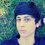# Geometry Problem

Try to solve this problem. If you could any one give the proof he's absolutely genius!! We have: Triangle ABC is isosceles at vertex A such that angle ABC = ACB = 80 degrees and BAC = 20. M is a point on AB such that AM = BC. Find the measure of MCA. Note: If you draw the figure and measured the angles you'll find the answer which is MCA = 10, CMA = 150 and CMB = 30. But need the proof Please?? Anyone??? Any genius Person?? ThanksNote by Yousef Kassir
6 years, 10 months ago

This discussion board is a place to discuss our Daily Challenges and the math and science related to those challenges. Explanations are more than just a solution — they should explain the steps and thinking strategies that you used to obtain the solution. Comments should further the discussion of math and science.

When posting on Brilliant:

• Use the emojis to react to an explanation, whether you're congratulating a job well done , or just really confused .
• Ask specific questions about the challenge or the steps in somebody's explanation. Well-posed questions can add a lot to the discussion, but posting "I don't understand!" doesn't help anyone.
• Try to contribute something new to the discussion, whether it is an extension, generalization or other idea related to the challenge.

MarkdownAppears as
*italics* or _italics_ italics
**bold** or __bold__ bold
- bulleted- list
• bulleted
• list
1. numbered2. list
1. numbered
2. list
Note: you must add a full line of space before and after lists for them to show up correctly
paragraph 1paragraph 2

paragraph 1

paragraph 2

[example link](https://brilliant.org)example link
> This is a quote
This is a quote
    # I indented these lines
# 4 spaces, and now they show
# up as a code block.

print "hello world"
# I indented these lines
# 4 spaces, and now they show
# up as a code block.

print "hello world"
MathAppears as
Remember to wrap math in $$ ... $$ or $ ... $ to ensure proper formatting.
2 \times 3 $2 \times 3$
2^{34} $2^{34}$
a_{i-1} $a_{i-1}$
\frac{2}{3} $\frac{2}{3}$
\sqrt{2} $\sqrt{2}$
\sum_{i=1}^3 $\sum_{i=1}^3$
\sin \theta $\sin \theta$
\boxed{123} $\boxed{123}$

Sort by:

Trig probably finishes this easily, but I present an elementary solution.

Construct points D and E on AB and AC respectively such that DE is parallel to BC, and angle EBC=60. Since triangle ABC is isosceles, angle DCB=angle EBC=60. Let BE and CD intersect at F and notice that BCF and DEF are equilateral. Now angle DCA = angle DAC = 20 which leads to DC=DA which is DM+DA=DF+CF=DF+BC which is DM=DF=DE. Since angle ADE = angle ABC = 80, angle DME = 50 since triangle DME is isosceles. Now DFM is isosceles too, with angle FDM=140, angle FMD=20, therefore triangle BFM is also isosceles, FM=BF=BC, therefore F is the circumcentre of BCM. Angle BMC is then half of angle BFC, therefore abgle BMC=30, angle CME=20, angle MCA=10.

- 6 years, 10 months ago

Look thanks v. v. v. v. v. v. v. very much for the answer :) Good job. But, What where is point G?? "...DC=DA which is DM+DA=DG+CG=DG+BC which is DM=DG=DE. Since angle DEA = 80, angle DME = 50 since triangle DME is isosceles...." this sentence i didn't understand. Thanks. And if you please explain it :)

- 6 years, 10 months ago

Woops G should be F. Just skipped over that, I'll fix it. DEA=BCA=80 because DE and BC are parallel, DME=50 since DME=DEM as DM=DE, and MDE=80. Actually DEA should be ADE, but they are both 80 anyway.

- 6 years, 10 months ago

Also it's imposibble for DM to equal DE, There's something strange! But thanks :) if you please send me another answer. Thanks. Because i need the answer badly

- 6 years, 10 months ago

Why impossible? Well a diagram speaks better, I used geogebra to draw this.

- 6 years, 10 months ago

I don't see the point of adding so many tags (most of which are unrelated to the topic).

- 6 years, 10 months ago

- 6 years, 10 months ago

Draw a line at 60° to AC & let it intersect with the perpendicular bisector of AM in N, the point being to the right of line AC. Now triangle MAN is isosceles with a base angles of 80° and an apex angle of 20°, with AN=MN. Further, Tr. MAN and Tr. ABC are congruent so AN=AC which makes Tr. CAN equilateral and /MNC=40° with MN=AN=NC or /NCA=70°. This means /_ACM=10° etc.

- 6 years, 9 months ago

Solution First of all, we can see that triangle BMC is isosceles. The measure of angle MBC is 80 (since M is on AB), which means that BMC and BCM are equal and they sum to 100, which means they both measure 50 degrees. The total measure of angle ACB is 80 degrees, so we subtract 50 to get 30. I think that your answer of 10 degrees is wrong.

- 6 years, 10 months ago

which means that BMC and BCM are equal

The problem stated AM=BC, not BM=BC.

- 6 years, 10 months ago

- 6 years, 10 months ago

let AM be x so BC is also x and BM is a-x ( a is equal side of isosceles). Apply sine rule in triangle ABC to find BC=x in terms of a so measure of AM is also found . Again applying sine rule in triangle AMC we can have aN equation in angle AMC . with some manipulation and trigo identities ANS-10

- 6 years, 10 months ago

hmm little difficult. but i will do it. tomorrow i will post the solution.

- 6 years, 10 months ago

(Y) Good luck then :)

- 6 years, 9 months ago

I believe there are various ways to tackle this problem, such as trig, transformations, or adding various points or lines. In my case I just create equilateral triangles to equate sides and stuff.

- 6 years, 10 months ago